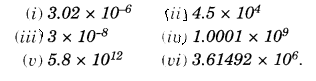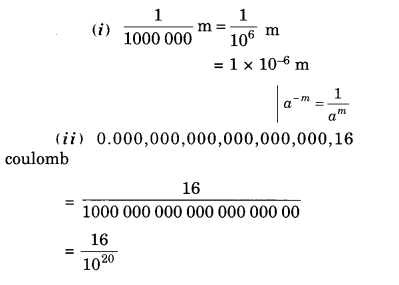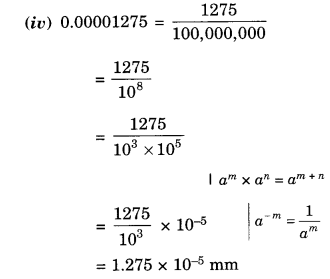NCERT Solutions for Class 8 Maths Chapter 12 Exponents and Powers Ex 12.2 are part of NCERT Solutions for Class 8 Maths. Here we have given NCERT Solutions for Class 8 Maths Chapter 12 Exponents and Powers Ex 12.2.

 Board CBSE Textbook NCERT Class Class 8 Subject Maths Chapter Chapter 12 Chapter Name Exponents and Powers Exercise Ex 12.2 Number of Questions Solved 4 Category NCERT Solutions

## NCERT Solutions for Class 8 Maths Chapter 12 Exponents and Powers Ex 12.2

Question 1.
Express the following numbers in standard form:
(i) 0.0000000000085
(ii) 0.00000000000942
(iii) 6020 000 000 000 000
(iv) 0.00000000837
(v) 31860000000
Solution.Question 2.
Express the following numbers in usual form:Solution.Question 3.
Express the number appearing in the following statements in standard form:
(i) 1 micron is equal to $$\frac { 1 }{ 1000000 }$$m.
(ii) Charge of an electron is 0.000,000,000,000,000,000,16 coulomb.
(iii) Size of a bacteria is 0.0000005 m
(iv) Size of a plant cell is 0.00001275 m
(v) Thickness of a thick paper is 0.07 mm.
Solution.Question 4.
In a stack there are 5 books each of thickness 20 mm and 5 paper sheets each of thickness 0.016 mm. What is the total thickness of the stack ?
Solution.
Total thickness of books
= 5 x 20 mm = 100 mm
Total thickness of paper sheets
= 5 x 0.016 mm = 0.080 mm
∴ Total thickness of the stack
= Total thickness of books + Total thickness of paper sheets
= 100 mm + 0.080 mm
= (100 + 0.080) mm
= 100.080 mm
= 1.0008 x $${ 10 }^{ 2 }$$ mm.

We hope the NCERT Solutions for Class 8 Maths Chapter 12 Exponents and Powers Ex 12.2 help you. If you have any query regarding NCERT Solutions for Class 8 Maths Chapter 12 Exponents and Powers Ex 12.2, drop a comment below and we will get back to you at the earliest.